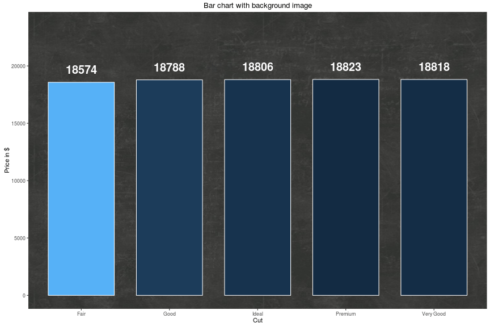Want to share your content on R-bloggers? click here if you have a blog, or here if you don't.

When producing so called infographics, it is rather common to use images rather than a mere grid as background. In this blog post, I will show how to use a background image with `ggplot2`.

## Packages required

The following code will install load and / or install the R packages required for this blog post.

```if (!require("pacman")) install.packages("pacman")
```

## Choosing the data

The data set I will be using in this blog post is named diamonds and part of the `ggplot2` package. It contains information about – surprise, surprise – diamonds, e.g. price and cut (Fair, Good, Very Good, Premium, Ideal). Using the `tapply`-function, we create a table returning the maximum prices per cut. Since we need the data to be organized in a data frame, we must transform the table using the `data.frame`-function.

```mydata <- data.frame(price = tapply(diamonds\$price, diamonds\$cut, max))
mydata\$cut <- rownames(mydata)
```
cut price
Fair 18574
Good 18788
Very Good 18818
Ideal 18806

## Importing the background image

The file format of the background image we will be using in this blog post is JPG. Since the image imitates a blackboard, we name it “blackboard.jpg”. The image file must be imported using the `readJPEG`-function of the `jpeg` package. The imported image will be saved into an object named `image`.

```imgage <- jpeg::readJPEG("blackboard.jpg")
```

To import other image file formats, different packages and functions must be used. The next code snippet shows how to import PNG images.

```image <- png::readPNG("blackboard.png")
```

## Drawing the plot

In the next step, we actually draw a bar chart with a backgriund image. To make blackboard.jpg the background image, we need to combine the `annotation_custom`-function of the `ggplot2` package and the `rasterGrob`-function of the `grid` package.

```ggplot(mydata, aes(cut, price, fill = -price)) +
ggtitle("Bar chart with background image") +
scale_fill_continuous(guide = FALSE) +
annotation_custom(rasterGrob(imgage,
width = unit(1,"npc"),
height = unit(1,"npc")),
-Inf, Inf, -Inf, Inf) +
geom_bar(stat="identity", position = "dodge", width = .75, colour = 'white') +
scale_y_continuous('Price in \$', limits = c(0, max(mydata\$price) + max(mydata\$price) / 4)) +
scale_x_discrete('Cut') +
geom_text(aes(label = round(price), ymax = 0), size = 7, fontface = 2,
colour = 'white', hjust = 0.5, vjust = -1)
```Using the specification `alpha = 0.5`, we add 50% opacity to the bars. `alpha` ranges between 0 and 1, with higher values indicating greater opacity.

```ggplot(mydata, aes(cut, price, fill = -price)) +
theme_neuropsychology() +
ggtitle("Bar chart with background image") +
scale_fill_continuous(guide = FALSE) +
annotation_custom(rasterGrob(imgage,
width = unit(1,"npc"),
height = unit(1,"npc")),
-Inf, Inf, -Inf, Inf) +
geom_bar(stat="identity", position = "dodge", width = .75, colour = 'white', alpha = 0.5) +
scale_y_continuous('Price in \$', limits = c(0, max(mydata\$price) + max(mydata\$price) / 4)) +
scale_x_discrete('Cut') +
geom_text(aes(label = round(price), ymax = 0), size = 7, fontface = 2,
colour = 'white', hjust = 0.5, vjust = -1)
```The recently published R package `neuropsychology` contains a theme named `theme_neuropsychology()`. This theme may be used to get bigger axis titles as well as bigger axis and legend text.

I hope you find this post useful and If you have any question please post a comment below. You are welcome to visit my personal blog Scripts and Statistics for more R tutorials.

Related Post